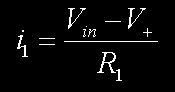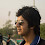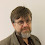## Tuesday 5 July 2011

### Gyrator analysisYour humble servant's article in this spring's number of SPRAT (pp 20 - 23), "A(nother) CW Filter", uses a circuit called a Gyrator to implement an inductive impedance. I was thinking ahead to a scheduled talk on the CW Filter at the Warrington club and realized it would be nice (that is to say "it would please me") to include an analysis of the gyrator.

This is a pretty standard circuit configuration, but a quick Google ((v) search the internet (for information) using the Google search engine) revealed that such analyses aren't exactly falling over one another - so here's the m0xpd story...

The circuit in question is shown below...The voltage at the non-inverting input to the op-amp is related to the input voltage by the potential divider equation:Ideal action of the op-amp (which is configured as a voltage follower) is summarised by:Substituting for the voltage at the non-inverting input gives (with apologies for the contribution of the "Microsoft Equation 3.0" equation editor):

The input impedance is the series combination of a resistor and a "virtual" inductor (in the sense that an inductor presents a positive reactance, proportional to frequency), with the inductor taking the value of the product of the two resistances and the capacitance...

1.2.